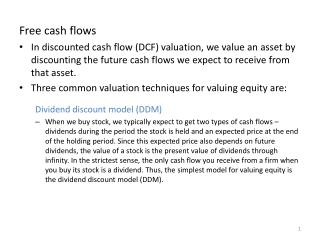# Free cash flows - PowerPoint PPT PresentationDownload PresentationFree cash flows

Free cash flowsDownload Presentation## Free cash flows

- - - - - - - - - - - - - - - - - - - - - - - - - - - E N D - - - - - - - - - - - - - - - - - - - - - - - - - - -
##### Presentation Transcript

1. Free cash flows • In discounted cash flow (DCF) valuation, we value an asset by discounting the future cash flows we expect to receive from that asset. • Three common valuation techniques for valuing equity are: Dividend discount model (DDM) • When we buy stock, we typically expect to get two types of cash flows – dividends during the period the stock is held and an expected price at the end of the holding period. Since this expected price also depends on future dividends, the value of a stock is the present value of dividends through infinity. In the strictest sense, the only cash flow you receive from a firm when you buy its stock is a dividend. Thus, the simplest model for valuing equity is the dividend discount model (DDM).

2. Discounting dividends is usually the most conservative estimate of value for the equity in any firm, since most firms pay out less in dividends than they can afford to. • The dividend cash flows are calculated as D = Net income*(1-Retention ratio) = Net income * Payout ratio

3. Free cash flows to equity (FCFE) • One problem that arises from using the DDM is that we may misvalue firms that consistently fail to return what they can afford to shareholders. • The FCFE is the cash flow that is left over after meeting all reinvestment needs and making debt payments. So, this cash flow could be paid out as dividends, and therefore will yield a more realistic value of the firm. • The FCFE are calculated as

4. Free cash flows to the firm(FCFF) • The FCFF is the cash flow to all holders of capital in the firm, i.e., the equity holders and the bond holders. • Done consistently, the FCFE and the FCFF should give the same value for equity. However, the FCFE are after net debt issues or payments and become more difficult to estimate when leverage is changing over time. FCFF does not have this problem since these are predebt cash flows. • The FCFE are calculated as

5. Notice that reinvestment needs have the opposite sign when we calculate free cash flows. Here’s why: • Free cash flows are essentially money that the firm can pay out to investors after reinvestment needs have been met. So: • Any capital expenditures are subtracted from the net income, since they represent cash outflows; while depreciation and amortization are added back in because they are non-cash charges. • Second, increases in working capital drain a firm’s cash flows, while decreases in working capital increase the cash flows available to investors. Since we are interested in the cash flow effects, we consider only changes in noncash working capital.

6. Sources: • Damodaran, Investment Valuation, 2nd ed. • Damodaran, Applied Corporate Finance, 3rd ed. • McKinsey & Company, Koller, Goedhardt, and Wessels, Valuation: Measuring and managing the value of companies, 5th ed.